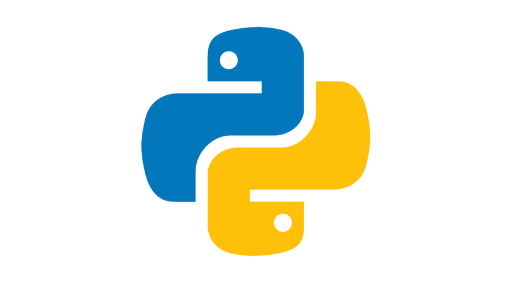# 字母大小写如何python3中用代码表示？```def decapitalize(string):
return str[:1].lower() + str[1:]

decapitalize('FooBar') # 'fooBar'
decapitalize('FooBar') # 'fooBar'```

```s = "programming is awesome"

print(s.title())
# Programming Is Awesome```

```def ReverseArray(ch):
lens=len(ch)
begin=0
end=lens-1
while begin<end:
while ch[begin]>='a' and ch[end]<='z' and end>begin:
begin+=1
while ch[begin]>='A' and ch[end]<='Z' and end>begin:
end-=1
ch[begin],ch[end]=ch[end],ch[begin]
if __name__=='__main__':
ch=list('AbcDef')
ReverseArray(ch)
i=0
while i<len(ch):
print(ch[i])
i+=1

f
b
c
e
D
A```